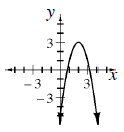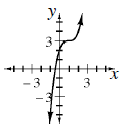### Home > INT3 > Chapter 11 > Lesson 11.1.4 > Problem11-47

11-47.

Sketch a graph of each equation below.

1. $y = −2\left(x − 2\right)^{2} + 3$1. $y = \left(x − 1\right)^{3} + 3$